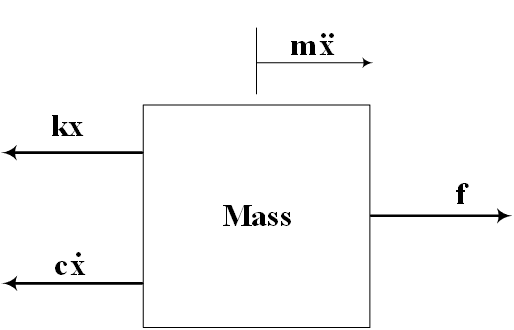## Welcome to the World of Modelling and Simulation

### What is Modelling?

This blog is all about system dynamics modelling and simulation applied in the engineering field, especially mechanical, electrical, and ...### Bond Graph Modelling, A Quick Learning: Part 2

This is a continuation from part-1 of the bond graph modelling. The first part discusses mostly about what the bond graph is, what are the components to build a model, where it is used, what are the advantages choosing this particular method, and so on, in a philosophical way. Here, in this part, I would like to jump in to show an example first before things get mundane.

Let us consider a very common mass-spring-damper system used in engineering analysis. Fig. 1 illustrates a typical mass-spring-damper system. One ends of the spring and damper are attached to the mass and other ends are connected to the fixed wall. It is considered that a force (f) is applied on the mass (m) causes its displacement (x) and two reaction forces such as spring force (stiffness, k) and damping force (damping coefficient, c) oppose the external force (f) simultaneously.Fig. 1: A typical mass-spring-damper system
With this information at hand, it is easy to derive differential equation by sketching the free body diagram. The free body diagram is shown in Fig. 2. The differential equation then may be obtained from Fig. 2 using Newton’s law for translation as follows:Fig. 2: Free body diagram of the system

So, we see that from sketching a free body diagram of the system and applying Newton's law, we are able to obtain the differential equation for this particular system. Now, with bond graph, we do not need any free body diagram, nor we need to think about the laws of classical mechanics. We follow a different approach which may be known as energy balance or conservation of energy method. The same differential equations may be obtained by drawing the following flow diagram which is essentially a bond graph model of the system.Fig. 3: Bond graph representation of the system

Fig. 3 shows some of the fundamental elements of the bond graph connected through the 1 and 0 junctions. For this simple mass-spring-damper system, the free body diagram approach looks simpler than the bond graph. Now imagine, when you are dealing with a very large system having many masses, springs and dampers connected in a very complex way - the derivation of differential equations manually is not feasible at all. Here comes the essence of the bond graph application. With a bond graph, we can accommodate many variables comfortably in a systematic way. In the next part, I will discuss elaborately how to draw the above bond graph for this simple system step by step in a plain language so that the readers might find this useful.